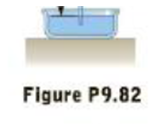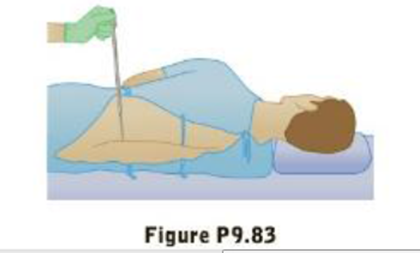Chapter 9, Problem 83AP

Chapter
Section
Textbook Problem

The human brain and spinal cord are immersed in the cerebrospinal fluid. The fluid is normally continuous between the cranial and spinal cavities and exerts a pressure of 100 to 200 mm of H2O above the prevailing atmospheric pressure. In medical work, pressures are often measured in units of mm of H2O because body fluids, including the cerebrospinal fluid, typically have nearly the same density as water. The pressure of the cerebrospinal fluid can be measured by means of a spinal tap. A hollow tube is inserted into the spinal column, and the height lo which the fluid rises is observed, as shown in Figure P9.83. If the fluid ruses to a height of 160. mm, we write its gauge pressure as 160. mm H2O. (a) Express this pressure in pascals, in atmospheres, and in millimeters of mercury. (b) Sometimes it is necessary to determine whether an accident victim has suffered a crushed vertebra that is blocking the flow of cerebrospinal fluid in the spinal column. In other cases, a physician may suspect that a tumor or other growth is blocking the spinal column and inhibiting the flow of cerebrospinal fluid. Such conditions ran be investigated by means of the Queckensted test. In this procedure, the veins in the patient’s neck are compressed lo make the blood pressure rise in the brain. The increase in pressure in the blood vessels is transmitted to the cerebrospinal fluid. What should be the normal effect on the height of the fluid in the spinal tap? (c) Suppose compressing the veins had no effect on the level of the fluid. What might account for this phenomenon?(a)

To determine
The pressure of the mercury in terms of Pascal’s, in atmospheres, and in millimeters of mercury.

Explanation

Given Info: Gauge pressure is 160mmH2O and Density of the water ρH2O is 103kg/m3 , Density of the mercury ρHg is 13.6×103kg/m3 .

Formula to calculate pressure of the mercury in terms of Pascal’s is,

Ppascals=gGpρH2O

• Ppascals is the pressure of the mercury,
• ρH2O is the density of the water,
• Gp is the gauge pressure,
• g is the  acceleration due to gravity.

Substitute 103kg/m3 for ρH2O , 9.8m/s2 for g and 160mmH2O for Gp to find Ppascals ,

Ppascals=(103kg/m3)(9.8m/s2)(160mm)(1m103mm)=(1568Pa)(1kPa103Pa)1.57kPa

Formula to calculate pressure of the mercury in terms of atmospheres is,

Patm=Ppascals1atm(1.013×105Pa)

• Ppascals is the pressure of the mercury in terms of Pascal’s,
• Patm is the pressure of the mercury in terms of atmospheres,

Substitute 1.57kPa for Ppascals to find Patm ,

Patm=1.57kPa(103Pa1kPa)(1atm(1.013×105Pa))=0

(b)

To determine
The normal effect on the height of the fluid in the spinal tap.

(c)

To determine
the compressing the veins had no effect on the level of the fluid.

Still sussing out bartleby?

Check out a sample textbook solution.

See a sample solution

The Solution to Your Study Problems

Bartleby provides explanations to thousands of textbook problems written by our experts, many with advanced degrees!

Get Started

In which domain and kingdom are humans classified?

Biology: The Dynamic Science (MindTap Course List)

If the airstream from a hair dryer is directed over a table-tennis ball, the ball can be levitated. Explain.

Physics for Scientists and Engineers, Technology Update (No access codes included)

How are holoplankton different from meroplankton?

Oceanography: An Invitation To Marine Science, Loose-leaf Versin